# Operators in Java

## Operators in Java with Examples

In this article, I am going to discuss the Operators in Java with Examples. Please read our previous article, where we discussed Type Casting in Java with Examples. At the end of this article, you will understand what are Java Operators and when and how to use them in Java Applications with examples.

##### What are Operators in Java?

An operator in Java is a symbol that is used to perform operations. Operators are the constructs that can manipulate the values of the operands. Consider the expression 2 + 3 = 5, here 2 and 3 are operands and + is called operator. In this article, the goal is to get you the expertise required to get started and work with operators in Java.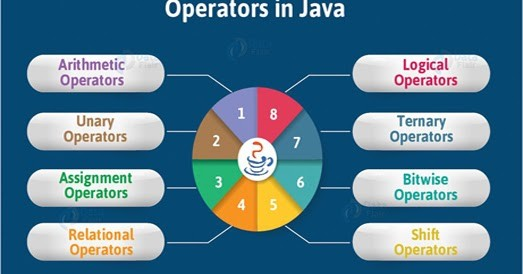There as many types of Operators in Java as follows:

• Arithmetic Operator
• Assignment Operator
• Relational Operator
• Logical Operator
• Bitwise Operator
• Unary Operator
• Shift Operator
• Ternary Operator
##### Arithmetic Operators in Java:

Arithmetic operators are used to perform mathematical operations like addition, subtraction, multiplication, etc. The following table shows the Types of Arithmetic Operators in Java:

 Operator Symbol Description Example Addition Operator + Adds the left operand with the right operand and returns the result. int a=10; int b=5; int c = a=b; //15 Subtraction Operator – Subtracts the left operand and right operand and returns the result. int a=10; int b=5; int c = a-b; //5 Multiplication Operator * It multiples the left and right operand and returns the result. int a=2; int b=3; int c=a*b; //6 Division Operator / Divides the left operand with the right operand and returns the result. int a=10; int b=5; int c=a/b; //0 Modulo Operator % Divides the left operand with the right operand and returns the remainder. int a=10; int b=5; int c=a/b; //2
##### Example to Understand Arithmetic Operators in Java:
```package Demo;
public class ArithmeticOperator
{
public static void main (String[]args)
{
int A = 10;
int B = 20;
System.out.println (A + B);
System.out.println (A - B);
System.out.println (A * B);
System.out.println (A / B);
System.out.println (A % B);
}
}```
###### Output: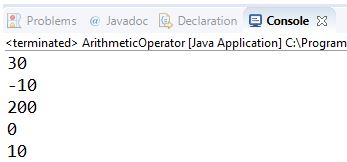##### Assignment Operators in Java:

The Java assignment operator is used to assign the value on its right to the operand on its left. The following table shows the Types of Assignment Operators in Java.

 Symbol Description Example = Assign the right operand to the left operand. int a=10;   int b=20;   a+=4; //a=a+4 (a=10+4)   b-=4; //b=b-4 (b=20-4) += Adding left operand with right operand and then assigning it to variable on the left. int a=5; a += 5; //a=a+5; -= subtracting left operand with right operand and then assigning it to variable on the left. int a=5; a -= 5; //a=a-5; *= multiplying left operand with right operand and then assigning it to the variable on the left. int a=5; a *= 5; //a=a*5; /= dividing left operand with right operand and then assigning it to a variable on the left. int a=5; a /= 5; //a=a/5; %= assigning modulo of left operand with right operand and then assigning it to the variable on the left. int a=5; a /= 5; //a=a/5;
##### Example to Understand Assignment Operators in Java:
```package Demo;
public class AssignmentOperator
{
public static void main (String[]args)
{
int a = 10;
int b = 20;
int c;
System.out.println (c = a);	// Output =10
System.out.println (b += a);	// Output=30
System.out.println (b -= a);	// Output=20
System.out.println (b *= a);	// Output=200
System.out.println (b /= a);	// Output=2
System.out.println (b %= a);	// Output=0
System.out.println (b ^= a);	// Output=0
}
}
```
###### Output: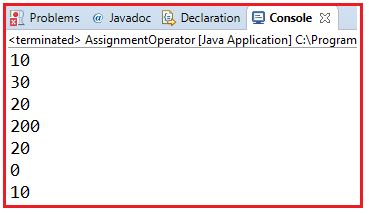##### Relational Operators in Java:

The Relational Operators in Java are also known as Comparison Operators. It determines the relationship between two operands and returns the Boolean results, i.e. true or false after the comparison. The following table shows the Types of Relational Operators in Java.

 Operator Symbol Description Example Equal to == returns true of the left-hand side is equal to the right-hand side. 5==3 is evaluated to be false. Not Equal to != returns true of the left-hand side is not equal to the right-hand side. 5!=3 is evaluated to be true. Less than < returns true of the left-hand side is less than the right-hand side. 5<3 is evaluated to false Less than or equal to <= returns true of the left-hand side is less than or equal to the right-hand side. 5<=5 is evaluated to be true Greater than > returns true of the left-hand side is greater than the right-hand side. 5>3 is evaluated to be true Greater than or Equal to >= returns true of the left-hand side is greater than or equal to the right-hand side. 5>=5 is evaluated to be true Instance of Operator instanceOf It compares an object to a specified type. String test = “asdf”; boolean result; result = test instanceOf String; //true
##### Example to Understand Relational Operators in Java:
```package Demo;
public class RelationalOperator
{
public static void main (String[]args)
{
int a = 10;
int b = 20;
System.out.println (a == b);	// returns false because 10 is not equal to 20
System.out.println (a != b);	// returns true because 10 is not equal to 20
System.out.println (a > b);	// returns false
System.out.println (a < b);	// returns true
System.out.println (a >= b);	// returns false
System.out.println (a <= b);	// returns true
}
}
```
###### Output: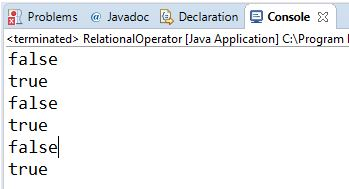##### Logical Operators in Java:

The Logical Operators in Java are mainly used in conditional statements and loops for evaluating a condition. They are basically used with binary numbers. The following table shows the types of Logical Operators in Java.

 Operator Symbol Description Example Logical OR || It returns true if either of the Boolean expressions is true. false || true is evaluated to true Logical AND && It returns true if all the Boolean Expressions are true false && true is evaluated to false.
##### Example to Understand Logical Operators in Java:
```package Demo;
public class LogicalOperators
{
public static void main (String[]args)
{
int a = 10;
System.out.println (a < 10 & a < 20);	 //returns false
System.out.println (a < 10 || a < 20);	 //returns true
System.out.println (!(a < 10 & a < 20)); //returns true
}
}```
###### Output: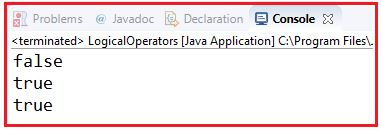##### Bitwise Operators in Java:

The Bitwise Operators in Java perform bit-by-bit processing. They can be used with any of the integer types. The following table shows the Types of Bitwise Operators in Java.

 Operator Symbol Description Example Bitwise OR | It compares corresponding bits of two operands. If either of the bits is 1, it gives 1. If not, it gives 0. int a=12, b=25; int result = a|b; //29 Bitwise AND & It compares corresponding bits of two operands. If either of the bits is 1, it gives 1. If either of the bits is not 1, it gives 0. int a=12, b=25; int result = a&b; //8 Bitwise XOR ^ It compares corresponding bits of two operands. If corresponding bits are different, it gives 1. If corresponding bits are the same, it gives 0. int a=12, b=25; int result = a^b; //21 Bitwise Complement ~ It inverts the bit pattern. It makes every 1 to 0 and every 0 to 1. int a=35; int result = ~a; //-36
##### Example to Understand Bitwise Operators in Java:
```package Demo;
public class BitwiseOperators
{
public static void main (String[]args)
{
int a = 58;			//111010
int b = 13;			//1101
System.out.println (a & b);	//returns 8 = 1000
System.out.println (a | b);	//63=111111
System.out.println (a ^ b);	//55=11011
System.out.println (~a);	//-59
}
}```
###### Output: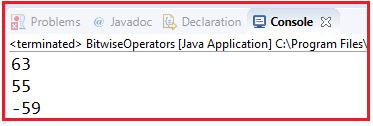##### Unary Operators in Java:

The Unary Operators in Java need only one operand. They are used to increment, decrement, or negate a value. The following table shows the Types of Unary Operators in Java.

 Operator Symbol Description Example Unary minus – It is used for negating the values. int a=5.2; int b = -a; //-5.2 Unary plus + It is used for giving positive values. int a=5.2; int b = +a; //5.2 Increment Operator ++ It is used for incrementing the value by 1. int a=5.2; int b = ++a; //6.2 Decrement Operator — It is used for decrementing the value by 1. int a=5.2; int b = –a; //4.2 Logical NOT Operator ! It is used for inverting a Boolean value boolean a=false; boolean b=!a; //true

Note: There are basically two types of Increment Operator:

##### Post Increment Operator in Java:

Value is first used for computing the result and then incremented.
Example:

int b = 10, c = 0;
// c=b then b=b+1
c=b++;
System.out.println(“Value of c (b++) = “ +c);

Output: Value of c (b++) = 10

##### Pre Increment Operator in Java:

Value is incremented first and then result is computed.
Example:

int a = 20, c = 0;
// a = a+1 and then c = a;
c=++a;
System.out.println(“Value of c (++a) = “ +c);

Output: Value of c (++a) = 21

Note: There are two types of Decrement Operator:

##### Post Decrement Operator in Java:

Value is first used for computing the result and then decremented.
Example:

int e = 40, c = 0;
// c=e then e=e-1
c=e–;
System.out.println(“Value of c (e–) = “ +c);

Output: Value of c (e–) = 40

##### Pre Decrement Operator in Java:

Value is decremented first and then result is computed.
Example:

int d = 20, c = 0;
// d=d-1 then c=d
c=–d;
System.out.println(“Value of c (–d) = “ +c);

Output: Value of c (–d) = 19

##### Example to Understand Unary Operators in Java:
```package Demo;
public class UnaryOperator
{
public static void main (String[]args)
{
int a = 10;
boolean b = true;
System.out.println (a++);	//returns 11
System.out.println (++a);
System.out.println (a--);
System.out.println (--a);
System.out.println (!b);	// returns false
}
}```
###### Output: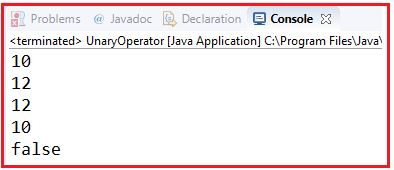##### Shift Operator in Java:

The Shift Operator in Java is used when you’re performing logical bits operations, as opposed to mathematical operations. These operators are used to shift the bits of a number left or right thereby multiplying or dividing the number by two respectively. They can be used when we have to multiply or divide a number by two.

##### Types of Shift Operators in Java:
 Operator Symbol Description Example Left Shift Operator << It is used to shift all of the bits in value to the left side of a specified number of times. 10<<2 //10*2^2=10*4=40 Right Shift Operator >> It is used to move the left operand’s value to the right by the number of bits specified by the right operand. 10>>2 //10/2^2=10/4=2
##### Example to Understand Shift Operators in Java:
```package Demo;
public class ShiftOperators
{
public static void main (String[]args)
{
int a = 58;
System.out.println (a << 2);	//232=11101000
System.out.println (a >> 2);	//returns 14=1110
System.out.println (a >>> 2);	//returns 14
}
}
```
###### Output: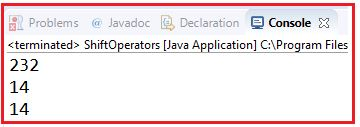##### Ternary Operator in Java:

The Ternary Operator in Java is also known as the Conditional Operator. It is the shorthand of the if-else statement. It is the one-liner replacement of the if-else statement in Java. It is called ternary because it has three operands. The general format of the ternary operator is: Condition ? if true : if false

The above statement means that if the condition evaluates to true, then execute the statements after the ‘?’ else execute the statements after the ‘:’.

##### Example:

int a=2;
int b=5;
int c = (a<b)?a:b;

Output: 2

##### Example to Understand Ternary Operator in Java:
```package Demo;
public class TernaryOperators
{
public static void main (String[]args)
{
int a = 20, b = 10, c = 30, res;
res = ((a > b) ? (a > c) ? a : c : (b > c) ? b : c);
System.out.println ("Max of three numbers = " + res);
}
}```

Output: Max of three numbers = 30

##### Operator Precedence and Associativity in Java

Java has well-defined rules for specifying the order in which the operators in an expression are evaluated when the expression has several operators. For example, multiplication and division have higher precedence than addition and subtraction. Precedence rules can be overridden by explicit parentheses.

Precedence and associative rules are used when dealing with hybrid equations involving more than one type of operator. In such cases, these rules determine which part of the equation to consider first as there can be many different valuations for the same equation.

##### Precedence order:

When two operators share an operand the operator with the higher precedence goes first. For example, 1 + 2 * 3 is treated as 1 + (2 * 3), whereas 1 * 2 + 3 is treated as (1 * 2) + 3 since multiplication has a higher precedence than addition.

##### Associativity:

When an expression has two operators with the same precedence, the expression is evaluated according to its associativity. For example x = y = z = 17 is treated as x = (y = (z = 17)), leaving all three variables with the value 17, since the = operator has right-to-left associativity (and an assignment statement evaluates to the value on the right hand side). On the other hand, 72 / 2 / 3 is treated as (72 / 2) / 3 since the / operator has left-to-right associativity. Some operators are not associative: for example, the expressions (x <= y <= z) and x++– are invalid.

The table below shows all Java operators from highest to lowest precedence, along with their associativity.

 Level Operator Description Associativity 16 [] . () access array element access object member parentheses left to right 15 ++ — unary post-increment unary post-decrement not associative 14 ++ — + – ! ~ unary pre-increment unary pre-decrement unary plus unary minus unary logical NOT unary bitwise NOT right to left 13 () new cast object creation right to left 12 * / % multiplicative left to right 11 + – + additive string concatenation left to right 10 << >> >>> shift left to right 9 < <= > >= instanceof relational not associative 8 == != equality left to right 7 & bitwise AND left to right 6 ^ bitwise XOR left to right 5 | bitwise OR left to right 4 && logical AND left to right 3 || logical OR left to right 2 ?: ternary right to left 1 =   +=   -= *=   /=   %= &=   ^=   |= <<=  >>= >>>= assignment right to left

Note: Larger the number means higher the precedence.